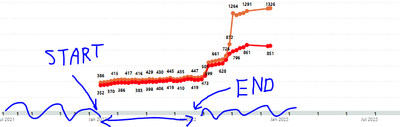cancel
Showing results for
Did you mean:Regular Visitor

## DAX measure - fixed line between two dates

Hello everyone,

Is there any way to force DAX to calculate a constatnt line (1) between two dates? Chart below:My current measure:*Measure "Test1" is added to chart as line

Every time  I play around with filter context (DIM_DATE) I receive a value 1 across all dates.
Thanks for any help 🙂

1 ACCEPTED SOLUTIONSuper User

HI @Filip_analyst

``````Test2 =
VAR date1 =
MAX ( DIM_DATE[DATE] )
VAR date2 =
DATE ( 2022, 01, 05 )
VAR date2 =
DATE ( 2022, 06, 10 )
RETURN
IF ( date1 >= date2 && date1 <= date3, 1 )``````
2 REPLIES 2Super User

HI @Filip_analyst

``````Test2 =
VAR date1 =
MAX ( DIM_DATE[DATE] )
VAR date2 =
DATE ( 2022, 01, 05 )
VAR date2 =
DATE ( 2022, 06, 10 )
RETURN
IF ( date1 >= date2 && date1 <= date3, 1 )``````Regular Visitor

Works as expected, thank you @tamerj1  ! 🙂